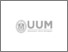UUM Electronic Theses and Dissertation
UUM ETD | Universiti Utara Malaysian Electronic Theses and Dissertation
FAQs | Feedback | Search Tips | Sitemap

# Block methods for direct solution of higher order ordinary differential equations using interpolation and collocation approach

Olusola, Kuboye John (2015) Block methods for direct solution of higher order ordinary differential equations using interpolation and collocation approach. PhD. thesis, Universiti Utara Malaysia.Preview
Text
s95332_01.pdf

## Abstract

Countless problems in real life situations involve rates of change of one or more independent variables. These rates of change can be expressed in terms of derivatives which lead to differential equations. Conventionally, initial value problems of higher order ordinary differential equations are solved by first reducing the equations to their equivalent systems of first order ordinary differential equations. Then, suitable existing numerical methods for first order ordinary
differential equations will be employed to solve the resulting equations. However, this approach will enlarge the equations and thus increases computational burden which may jeopardise the accuracy of the solution. In overcoming the setbacks, direct methods were proposed. Disappointedly, most of the existing direct methods
approximate the numerical solution at one point at a time. Block methods were then introduced with the aim of approximating numerical solutions at many points concurrently. Several new block methods using interpolation and collocation approach for solving initial value problems of higher order ordinary differential equations directly were developed in this study to increase the accuracy of the solution. In developing these methods, a power series was used as an approximate solution to the problems of ordinary differential equations of order d. The power series was interpolated at d points before the last two points while its highest
derivative was collocated at all grid points in deriving the new block methods. In addition, the properties of the new methods such as order, error constant, zerostability, consistency, convergence and region of absolute stability were also
investigated. The developed methods were then applied to solve several initial value problems of higher order ordinary differential equations. The numerical results indicated that the new methods produced better accuracy than the existing methods
when solving the same problems. Therefore, this study has successfully produced new methods for solving initial value problems of higher order ordinary differential equations.

Item Type: Thesis (PhD.) Omar, Zurni 5789 Interpolation, collocation, block method, direct solution, higher order initial value problems. Q Science > QA Mathematics > QA273-280 Probabilities. Mathematical statistics Awang Had Salleh Graduate School of Arts & Sciences 20 Jul 2016 10:17 20 Jul 2016 10:17 Awang Had Salleh Graduate School of Arts and Sciences Omar, Zurni https://etd.uum.edu.my/id/eprint/5789